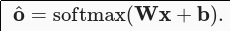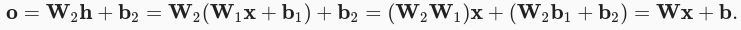# More On Multi Layer Perceptron

If you are looking for a really simple understanding of Deep Neural Nets in installments of tiny nuggets of information you are in the right place. For any competent practical material on the topic please refer to Dive Into Deep Learning. I think the material there in general is very practical and can be followed by newbies through experts alike.

### Why Hidden Layers

If Neural Architectures only correlated inputs to the outputs using just linear transformations then the resulting Neural Networks would be called Linear Neural Networks (LNNs). For the most part, Linear NNs are useful in cases where the inputs linearly determine output, ie., any changes to the inputs produce an output that can be correlated using . “linearity implies the weaker assumption of monotonicity”

The figure shows linear correlation between input and output, for example, when the output is a linear transformation of the input like below.Problems that are of the following nature can benefit from linear correlations:

• Likelihood of a individual paying the loan off with an input parameter being income (an increase here implies increase in the likelihood of paying the loan back => Linear).
• Correlation of a Body Temperature to Likelihood of Death

However, in more sophisticated problems such as Computer Vision, each input would something like a single pixel’s intensity. There are no plausible correlations of this single pixel intensity with the outcome prediction like if the image is not a hotdog. Clearly, there is no linear transformation between input and output that can solve this class of problems.

To generalize the previously shown Single Layer Perceptron to solve more wider class of probems, is to use several of such linear correlation layers feed from the output of another such Perceptron. The output from this Perceptron is further fed into the next and so on. This can be imagined as a stack of layers that consume input on one end, while passing the output to the next whose output is `hidden`. By stacking enough such layers we can design models to handle more general class of functions.#### Linear to NonLinear Correlation

This should make it clearer.In the near future, we will look into Activation Functions and such.